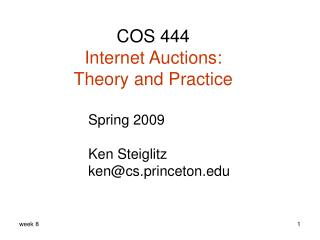Download PresentationCOS 444 Internet Auctions: Theory and Practice

Loading in 2 Seconds...

# COS 444 Internet Auctions: Theory and Practice - PowerPoint PPT Presentation

COS 444 Internet Auctions: Theory and Practice. Spring 2009 Ken Steiglitz ken@cs.princeton.edu. Some other auctions in A rs. All-pay with reserve. Consider simple case with n=2 and uniform iid values on [0,1]. We also choose the optimal v * = ½.I am the owner, or an agent authorized to act on behalf of the owner, of the copyrighted work described.
Download Presentation## COS 444 Internet Auctions: Theory and Practice

An Image/Link below is provided (as is) to download presentation

Download Policy: Content on the Website is provided to you AS IS for your information and personal use and may not be sold / licensed / shared on other websites without getting consent from its author.While downloading, if for some reason you are not able to download a presentation, the publisher may have deleted the file from their server.

- - - - - - - - - - - - - - - - - - - - - - - - - - E N D - - - - - - - - - - - - - - - - - - - - - - - - - -
Presentation Transcript
1. COS 444 Internet Auctions:Theory and Practice Spring 2009 Ken Steiglitz ken@cs.princeton.edu

2. Some other auctions in Ars All-pay with reserve Consider simple case with n=2 and uniform iid values on [0,1]. We also choose the optimal v* = ½. How do we choose b0 to achieve this v* ?

3. All-pay with reserve Standard argument: If your value is v*you win if and only if you have no rival bidder. (This is the point of indifference between bidding and not bidding, and your expected surplus is 0.) Therefore, bid as low as possible! Therefore, b(v*) = b0. And so b0 = v*2 = ¼ . Notice that this is an example where the reserve is not equal to the entry value v* .

4. Loser weeps auction, n=2, uniform v Winner gets item for free, loser pays his bid! Auction is in Ars. The expected payment is therefore and therefore, choosing v* = ½ as before (optimally), To find b0 , set E[surplus] = 0 at v = v* , and again argue that b(v*) = b0 . This gives us ( goes to ∞ !)

5. Santa Claus auction, n=2, uniform v • Winner pays her bid • Idea: give people their expected surplus and try to arrange things so bidding truthfully is an equilibrium. • Pay bidders • To prove: truthful bidding is a SBNE

6. Santa Claus auction, con’t Suppose 2 bids truthfully and 1 has value v and bids b. Then because F(b) = prob. winning. For equil.: □ (use reserve b0 = v* )

7. Matching auction: not in Ars • Bidder 1 may tender an offer on a house, b1 ≥ v* • Bidder 2 currently leases house and has the option of matching b1 and buying at that price. If bidder 1 doesn’t bid, bidder 2 can buy at v* if he wants to

8. Matching auction: not in Ars • To compare with optimal auctions, choose v*= ½; uniform iid IPV’s on [0,1] • Bidder 2’s best strategy: If 1 bids, match b1iff v2 ≥ b1 ; else bid ½ iff v2 ≥ ½ • Bidder 1 should choose b1 ≥ ½ so as to maximize expected surplus. This turns out to be b1 = ½ . To see this

9. Matching auction: not in Ars Choose v* = ½ for comparison. Bidder 1 tries to max (v1− b1 )·{ prob. 2 chooses not to match } = (v1 − b1 )·b1  b1 = 0 if v1 < ½ = ½ if v1 ≥½ □

10. Matching auction: not in Ars Notice: When ½ < v2 < v1 , bidder 2 gets the item, but values it less than bidder 1 inefficient! E[revenue to seller] turns out to be 9/24 (optimal in Ars is 10/24; optimal with no reserve is 8/24) BTW, …why is this auction not in Ars ?

11. Risk aversion Intuition: Suppose you care more about losses than gains. How does that affect your bidding strategy in SP auctions? First-price auctions? recall

12. Utility model

13. Risk aversion & revenue ranking Result: Suppose bidders’ utility is concave. Then with the assumptions of Ars , RFP ≥ RSP Proof: Let γbe the equilibrium bidding function in the risk-averse case, and β in the risk-neutral case.

14. Revenue ranking Let the bidder bid as if her value is z, while her actual value is x. In a first-price auction, her expected surplus is where W(z) = F(z)n-1 is the prob. of winning. As usual, to find an equilibrium, differentiate wrt z and set the result to 0 at z = x: where w(x) = W΄(x). where w(x) = W΄(x).

15. Revenue ranking In the risk neutral case this is just: The utility function is concave:

16. Revenue ranking Using this, Now we can see that γ΄(0) > β΄(0). If not, then there would be an interval near 0 where γ≤β, and then which would be a contradiction.

17. Revenue ranking Also, it’s clear that γ(0) = β(0) = 0. So γ starts out above βat theorigin. To show that it stays above β, consider what would happen should it cross, say at x = x* : A contradiction. □

18. Constant relative risk aversion (CRRA) Defined by utility In this case we can solve [MRS 03] for Very similar to risk-neutral form, but bid as if there were (n−1)/ρ instead of (n−1) rivals!